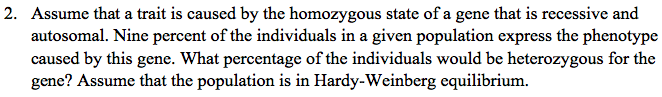# Problem: Assume that a trait is caused by the homozygous state of a gene that is recessive and autosomal. Nine percent of the individuals in a given population express the phenotype caused by this gene. What percentage of the individuals would be heterozygous for the gene? Assume that the population is in Hardy-Weinberg equilibrium.

###### FREE Expert Solution

For problems involving Hardy-Weinberg equilibrium, the relative frequency of the dominant allele is usually represented by p, and for the recessive allele, it is represented by q. Moreover, the genotypic frequencies can be expressed as p2 for homozygous dominant, q2 for the homozygous recessive, and 2pq for heterozygous.###### Problem Details

Assume that a trait is caused by the homozygous state of a gene that is recessive and autosomal. Nine percent of the individuals in a given population express the phenotype caused by this gene. What percentage of the individuals would be heterozygous for the gene? Assume that the population is in Hardy-Weinberg equilibrium.